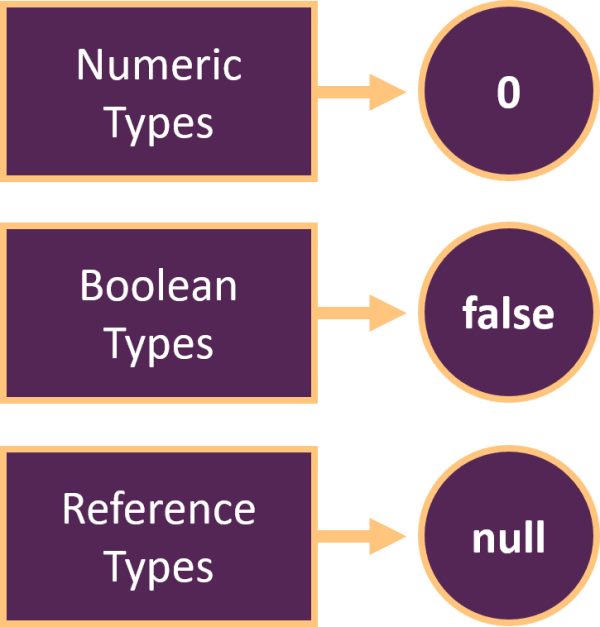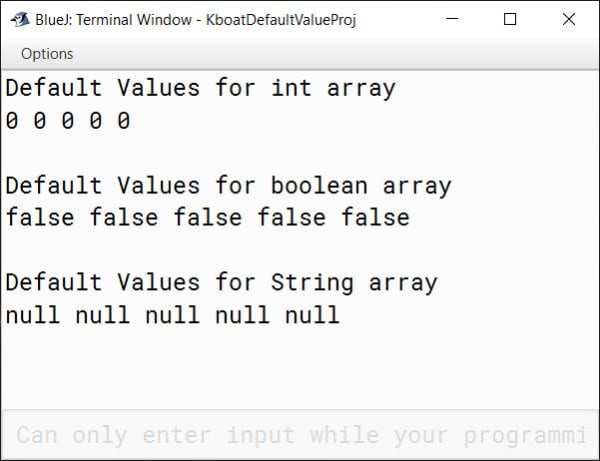# Single Dimensional Array: Creation with new Operator

## ICSE Computer Applications

To quickly recap, we declare and initialize a single dimensional array like this:

`int arr[] = {1, 2, 3, 4, 5, 6};`

General syntax for array declaration and initialization is this:

`<type> <array-name>[] = {<value1>, <value2>, ….};`

Length or size of the array is the number of elements present in the array.

Index or subscript of an array ranges from 0 to array length – 1.

The general syntax to access an individual element of an array is this:

`<array-variable>[<index>];`

For example, arr will give us 4 i.e. the fourth element of the array.

We can access the elements of the array with a loop like this:

```for (int i = 0; i < arr.length; i++) {
System.out.println(arr[i]);
}```

## Creating Array with new Operator

In this lesson, we will learn another way of creating a single dimensional array and accepting user provided data in it.

We first declare a single dimensional array like this:

`int arr[];`

The general syntax for array declaration is:

`type array-variable[];`

Start with the data type of the array, followed by the name of the array and then square brackets to tell the compiler that it is an array variable not a regular variable and finally end the statement with a semicolon.

After this, we use the new operator to create the array and assign it to `arr` like this:

`arr = new int;`

The general syntax for single dimensional array creation with new operator is:

`array-variable = new type[size];`

First write the array-variable then equal to sign followed by the new operator and the data type of the array. In this format, we need to explicitly provide the size or length of the array in square brackets. Finally, we end the statement with a semicolon.

To summarize, creating the array is a 2-step process:

```int arr[];
arr = new int;```

First declare the array, next use the new operator to create the array.

This first statement just tells the compiler that we intend to use `arr` as an int array. It doesn’t create any array. After the execution of this statement, `arr` contains null meaning nothing. Remember, I told you that you can think of an array as a row of numbered boxes. This first statement is just defining the name of this row of numbered boxes. The actual boxes are not created yet.

The next statement creates these boxes using the new operator. We will look at the new operator in more detail when we will discuss classes. We tell the new operator, what type of boxes we need and how many we need through the type and size, respectively.

After the execution of these statement, we have created an int array of six elements. We can access its individual elements like before using the subscripted variable and subscript.

## Default Values

When we create an array using the new operator, Java automatically assigns a default value to all the elements in the array. Default values for different data types are different.It is zero for numeric types, false for boolean types and null for reference types.

Below program shows default values for arrays of different data types:

```public class KboatDefaultValue
{
public static void main(String args[]) {

int integerArray[] = new int;        //Numeric Type
boolean boolArray[] = new boolean;   //Boolean Type
String stringArray[] = new String;   //Reference Type

System.out.println("Default Values for int array");
for (int i = 0; i < 5; i++) {
System.out.print(integerArray[i] + " ");
}

System.out.println("\n\nDefault Values for boolean array");
for (int i = 0; i < 5; i++) {
System.out.print(boolArray[i] + " ");
}

System.out.println("\n\nDefault Values for String array");
for (int i = 0; i < 5; i++) {
System.out.print(stringArray[i] + " ");
}
}
}```

In the program above, I have created an int, boolean and String array. Size of each of these arrays is 5. After that I am printing all the elements of these arrays.As you see in the output, all elements of int array are zero, boolean array are false and String array are null.

## Combining Array Declaration and Creation

Coming back to array declaration and creation, we learnt that we can declare and create an array using these two statements:

```int arr[];
arr = new int;```

We can also combine the declaration and creation of the array into a single statement like this:

`int arr[] = new int;`

Most of the times we will use this way to declare and create an array. One more important thing, we can use a variable also to specify this size like this:

```int size = 6;
int arr[] = new int[size];```

## Assigning Values to Array Elements

We have created the array but all the elements in the array are zero. Let’s assign some values to the array elements.

We assign value to an array element like this `arr = 1;`. This will assign the value of 1 to the first element of the array and this `arr = 2;` will assign the value of 2 to the second element of the array. Remember from the last lecture, the index of array starts at 0 not 1.

Similarly, we can assign values to other elements of the array:

```arr = 3;
arr = 4;
arr = 5;
arr = 6;```